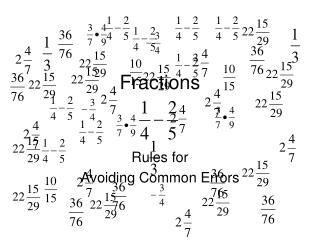DownloadDownload PresentationFractions

# Fractions

Télécharger la présentation## Fractions

- - - - - - - - - - - - - - - - - - - - - - - - - - - E N D - - - - - - - - - - - - - - - - - - - - - - - - - - -
##### Presentation Transcript

1. Fractions Rules for Avoiding Common Errors

2. Simplifying Reduce = = = 10 = Greatest Common Factor Write the numerator and denominator as a product of factors, then cancel common factors and obtain the result.

3. Simplifying = = = = Write the numerator and denominator as a product of common factors then cancel the common factors and obtain the result. Repeat the simplification process until all common factors are removed.

4. Converting Mixed Numbers 1 1 5 5 3 3 3 3 + 16 = 3 Multiply the whole number by the denominator Add the numerator to the result Place over the initial denominator Compute the final result

5. Converting Mixed Numbers 2 2 6 6 9 9 9 9 + 56 = 9 Multiply the whole number by the denominator Add the numerator to the result Place over the initial denominator Compute the final result

6. 3 10 2 5 15 8 11 44 Multiplying 3 = = = 11 4 2 Fraction Multiplication Fraction Simplification

7. 2 15 3 5 5 2 3 4 2 2 Multiplying 2 = = = 3 Fraction Multiplication Fraction Simplification

8. Fraction Division Dividing 3 3 12 12 28 7 3 4 = = = 3 5 7 7 5 5 5 Fraction Simplification and Multiplication

9. Fraction Division 4 8 Dividing 4 = 5 15 8 8 24 24 3 5 3 8 = = 8 5 15 15 5 5 9 = = 9 1 Fraction Simplification Fraction Multiplication Mixed Number Conversion

10. Fraction Addition/Subtraction Adding/Subtracting Fraction Simplification 3 3 1 1 2 1 - - = = = 8 8 8 8 8 4 8

11. 11 11 8 28 28 28 19 11 + 8 28 Fraction Addition/Subtraction Adding/Subtracting 11 2 2 4 = + + + = 28 7 7 4 = = 28 28 Common Denominator = ??????

12. Rules of Fractions Review • Fraction Simplification • Mixed Number Conversion • Fraction Multiplication • Fraction Division • Fraction Addition/Subtraction

13. 21 13 8 45 5 6 16 5 YOU TRY IT

14. Fractions: Steps for Success • Know the fraction rules and how to apply them • Show your work and write out each step • Check your work for errors or careless mistakes

15. 19 1 = + 8 4 19 2 = + 8 8 21 = 8 Mixed Number Conversion Fraction Addition Fraction Addition

16. 25 12 = - 45 45 13 45 Fraction Subtraction = Fraction Subtraction

17. 3 3 4 = 2 3 5 3 4 = 2 5 2 3 2 = 2 5 3 2 = 1 5 6 = 5 Fraction Simplification Fraction Simplification Fraction Simplification Fraction Simplification Fraction Multiplication

18. 5 3 = 12 4 5 3 = 3 4 4 5 1 = 4 4 5 = 16 Fraction Division Fraction Simplification Fraction Simplification Fraction Multiplication